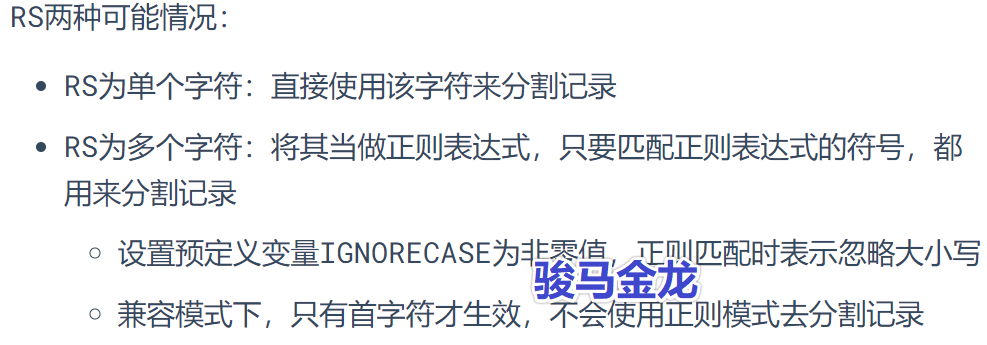# 精通awk系列(7)：awk读取行的细节

## 详细分析awk如何读取文件

awk读取输入文件时，每次读取一条记录(record)(默认情况下按行读取，所以此时记录就是行)。每读取一条记录，将其保存到$0中，然后执行一次main代码段。 awk '{print$0}' a.txt

touch x.log  # 创建一个空文件
awk '{print "hello world"}' x.log

# RS="\n" 、 RS="m"
awk 'BEGIN{RS="\n"}{print $0}' a.txt awk 'BEGIN{RS="m"}{print$0}' a.txt

RS通常设置在BEGIN代码块中，因为要先于读取文件就确定好RS分隔符。

RS指定输入记录分隔符时，所读取的记录中是不包含分隔符字符的。例如RS="a"，则$0中一定不可能出现字符a。特殊的RS值用来解决特殊读取需求： • RS=""：按段落读取 • RS="\0"：一次性读取所有数据，但有些特殊文件中包含了空字符\0 • RS="^$"：真正的一次性读取所有数据，因为非空文件不可能匹配成功
• RS="\n+"：按行读取，但忽略所有空行

# 按段落读取：RS=''
$awk 'BEGIN{RS=''}{print$0"------"}' a.txt

# 一次性读取所有数据：RS='\0' RS="^$"$ awk 'BEGIN{RS='\0'}{print $0"------"}' a.txt$ awk 'BEGIN{RS='^$'}{print$0"------"}' a.txt

# 忽略空行：RS='\n+'
$awk 'BEGIN{RS='\n+'}{print$0"------"}' a.txt

# 忽略大小写：预定义变量IGNORECASE设置为非0值
$awk 'BEGIN{IGNORECASE=1}{print$0"------"}' RS='[ab]' a.txt  

awk 'BEGIN{RS="(fe)?male"}{print RT}' a.txt

## 两种行号：NR和FNR

• NR：所有文件的行号计数器
• FNR：是各个文件的行号计数器
awk '{print NR}' a.txt a.txt
awk '{print FNR}' a.txt a.txt
posted @ 2019-11-23 15:43  骏马金龙  阅读(...)  评论(...编辑  收藏